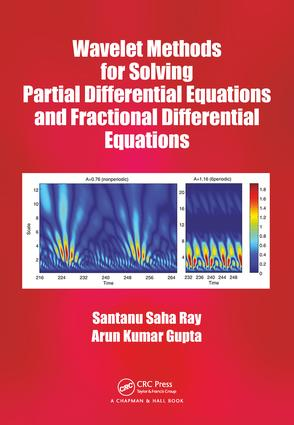# Wavelet Methods for Solving Partial Differential Equations and Fractional Differential Equations

## 1st Edition

Chapman and Hall/CRC

273 pages | 8 Color Illus. | 34 B/W Illus.

Hardback: 9781138053816
pub: 2018-01-31
\$155.00
x
eBook (VitalSource) : 9781315167183
pub: 2018-01-12
from \$28.98

FREE Standard Shipping!

### Description

The main focus of the book is to implement wavelet based transform methods for solving problems of fractional order partial differential equations arising in modelling real physical phenomena. It explores analytical and numerical approximate solution obtained by wavelet methods for both classical and fractional order partial differential equations.

Numerous Analytical and Numerical Methods. Introduction. Variational Iteration Method (VIM). First Integral Method (FIM). Homotopy Perturbation Method (HPM). Optimal Homotopy Asymptotic Method (OHAM). Homotopy Analysis Method (HAM). Haar Wavelets and the Operational Matrices. Legendre Wavelets. Chebyshev Wavelets. Hermite Wavelets.Numerical Solution of Partial Differential Equations by Haar Wavelet Method.

Introduction. Outline of Present Study. Application of Haar Wavelet Method to Obtain Numerical Solution of Burgers’ Equation. Haar Wavelet Based Scheme for Modified Burgers’ Equation. Analytical and Numerical Methods for Solving Burgers-Huxley Equation. Application of Analytical and Numerical Methods for Solving Huxley Equation

Numerical Solution of Generalized Modified KdV Equation. Error of Collocation Method. Error Analysis. Conclusion. Numerical Solution of System of Partial Differential Equations. Introduction. Overview of the Problem. Analytical Solution of System of Nonlinear PDEs. Convergence of HPM. Convergence of OHAM. Numerical Results and Discussion. A Numerical Approach to Boussinesq-Burgers’ Equations. Convergence of Haar Wavelet Approximation. Numerical Results. Conclusion. Numerical Solution of Fractional Differential Equations by Haar Wavelet Method. Introduction to Fractional Calculus. Fractional Derivative and Integration. Outline of the present study. Application of Analytical and Numerical Techniques to Fractional Burgers-Fisher Equation. Numerical Results for Fractional Burgers-Fisher Equation. Application of Analytical and Numerical Methods to Fractional Fisher’s Type Equation. Numerical Results for Fractional Fisher’s Equation. Solution of Fractional Fokker-Planck Equation. Numerical Results for Fractional Fokker-Planck Equation. Convergence Analysis of Two-dimensional Haar Wavelet Method. Conclusion.Application of Legendre Wavelet Methods for Numerical Solution of Fractional Differential Equations. Introduction. Outline of Present Study. Solution of time-Fractional Parabolic Partial Differential Equation. Numerical Results of Fractional order PDE. Implementation of Legendre Wavelets for Solving Fractionl KBK Equation. Numerical Results and Discussion of time-Fractiuonal KBK. Application of Analytical and Numerical Methods for Solving time-Fractional sKdV Equation. Numerical Results and Discussion of time-Fractiuonal sKdV Equation. Convergence of Legendre Wavelet. Solution of Fractional Kaup-Kupershmidt Equation Using Legendre Multiwavelets. Application of Analytical and Numerical Methods for Solving time-Fractional Kaup-Kupershmidt Equation. Numerical Results of Fractional Kaup-Kupershmidt Equation. Conclusion. Appliaction of Chebyshev Wavelet Methods for Numerical Simulation of Fractional Differential Equations. Introduction. Outline of Present Study. Formulation of Time-Fractional Sawada-Kotera Equation. Application of Analytical and Numerical Methods for Solving Fractional Sawada-Kotera Equation. Numerical Results of Fractional Sawada-Kaotera Equation. Application of Two-Dimensional Chebyshev Wavelet Method on time-Fractional Camassa-Holm Equation. Numerical Results and Discussion. Implemantation of Two-Dimensional Chebyshev Wavelet Method. For Approximate Solution of Riesz space-Fractional Sine-Gordon. Equation. Numerical Results and Discussion. Convergence Analysis of Chebyshev Wavelet. Conclusion. Appliaction of Hermite Wavelet Method for Numerical Simulation of Fractional Differential Equations. Introduction. Algorithm of Hermite Wavelet Method. Application of Analytical and Numerical Methods for Solving Nonlinear time-Fractional Modified Fornberg-Whitham Equation. Numerical Results and Discussion. Application of Analytical Methods to Determine the Exact Solutions of time-Fractional Modified Fornberg-Whitham Equation Numerical Results and Discussion. Application of Analytical and Numerical Methods for Solving time-Fractional Coupled Jaulent-Miodek Equations. Numerical Results and Discussion. Convergence of Hermite Wavelet. Conclusion. Implementation of Petrov-Galerkin Method for Solving FPDEs. Introduction. Implemantation of Petrov-Galerkin Method for Numerical Solution of time-Fractional KdV-Burgers Equation. Numerical Results and Discussion. Implemantation of Petrov-Galerkin Method for Numerical Solution of time-Fractional Sharma-Tasso-Olver Equation.Numerical Results and Discussion. Conclusion.

Dr. Santanu Saha Ray is an associate professor in the Department of Mathematics at the National Institute of Technology in Rourkela, India. He is a member of the Society for Industrial and Applied Mathematics and the American Mathematical Society. He is also the editor-in-chief of the International Journal of Applied and Computational Mathematics and the author of numerous journal articles and two books: Graph Theory with Algorithms and Its Applications: In Applied Science and Technology and Fractional Calculus with Applications for Nuclear Reactor Dynamics. His research interests include fractional calculus, mathematical modeling, mathematical physics, stochastic modeling, integral equations, and wavelet transforms. Dr. Saha Ray earned his PhD from Jadavpur University.